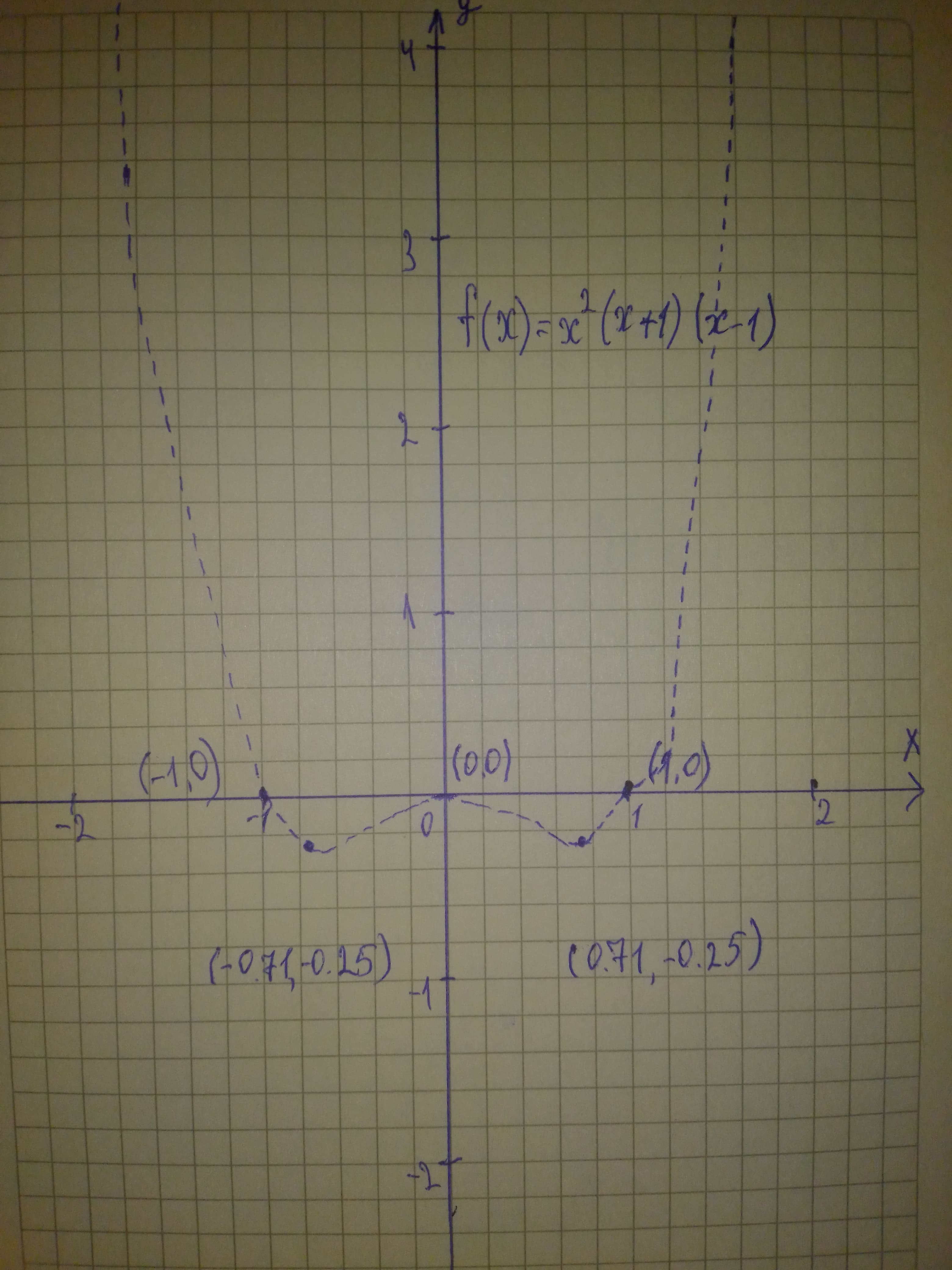# Graph each polynomial function. Factor first if the expression is not in factored form. f(x)=x^{2}(x+1)(x-1)Maiclubk 2021-01-22 Answered
Graph each polynomial function. Factor first if the expression is not in factored form.
$f\left(x\right)={x}^{2}\left(x+1\right)\left(x-1\right)$
You can still ask an expert for help

• Questions are typically answered in as fast as 30 minutes

Solve your problem for the price of one coffee

• Math expert for every subject
• Pay only if we can solve itaprovard

$f\left(x\right)={x}^{2}\left(x+1\right)\left(x-1\right)$ The function in factored form
The function has three zeros 0, -1, and 1
So, the graph of f(x) crosses the x-axis at (0,0), (-1,0), and (1,0)
To find the y-intercept, substitute 0 for x in f(x)
$f\left(x\right)={x}^{2}\left(x+1\right)\left(x-1\right)$
$f\left(0\right)={\left(0\right)}^{2}\left(0+1\right)\left(0-1\right)$ Substitute 0 for x
$=0$
So, the function f(x) crosses the y-axis at (0,0)
${x}^{2}\cdot x\cdot x={x}^{4}$
Since the leading coefficient is positive and the function f(x) of degree 4 (even degree)
So, the end behavior is
$x\to \mathrm{\infty },f\left(x\right)\to \mathrm{\infty }$
$x\to -\mathrm{\infty },f\left(x\right)\to \mathrm{\infty }$
See the graph below